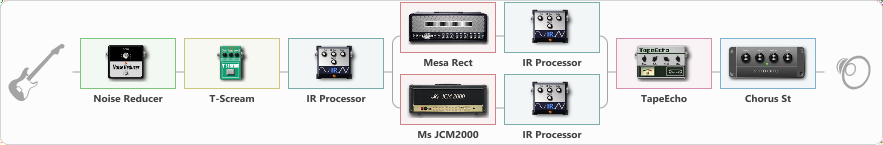# guitar 2ª

Discussion in 'ToneLib-GFX presets' started by carlinos3, Jun 17, 2021.

1. guitar 2ª

Preset name: boble guitarra

Effects chain:Effect: "Noise Reducer" (Dinámica / Filtro), active - "yes"
{
"Sens" = 88
"Mode" = Soft
}

Effect: "T-Scream" (Overdrive / Distorsión), active - "yes"
{
"Drive" = 22
"Tone" = 80
"Level" = 79
}

Effect: "IR Processor" (Gabinetes), active - "yes"
{
"IR" = pre-tonal_dc
"Low Cut (Hz)" = 250
"Hi Cut (kHz)" = 20.0
"Mix" = 59
"Level (dB)" = 20
}

Effect: "Splitter" (Dinámica / Filtro)
{
"A-Bypass" = Off
"A-Pan" = 100
"A-Level" = 55
"B-Bypass" = Off
"B-Pan" = -100
"B-Level" = 55

'A' branch:
{

{
"Gain" = 100
"Bass" = 50
"Middle" = 49
"Treble" = 50
"Presence" = 80
"Master" = 74
"Level (dB)" = 3
}

Effect: "IR Processor" (Gabinetes), active - "yes"
{
"IR" = charv.1_dc
"Low Cut (Hz)" = 0
"Hi Cut (kHz)" = 20.0
"Mix" = 100
"Level (dB)" = 2
}
}
'B' branch:
{

{
"Gain" = 100
"Bass" = 50
"Middle" = 50
"Treble" = 50
"Presence" = 50
"Master" = 68
"Level (dB)" = 4
}

Effect: "IR Processor" (Gabinetes), active - "yes"
{
"IR" = ibanez_dc
"Low Cut (Hz)" = 0
"Hi Cut (kHz)" = 20.0
"Mix" = 100
"Level (dB)" = 0
}
}
}

Effect: "TapeEcho" (Retrasar), active - "yes"
{
"Time" = 502
"Feedback" = 0
"Tone" = 50
"LoDamp" = 19
"Mix" = 48
}

Effect: "Chorus St" (Modulación / Sfx), active - "yes"
{
"Speed" = 1.9
"Depth" = 31
"Center" = 4.4
"Mix" = 40
}

Note: You will need to download and install the ToneLib-GFX software to use the preset.

#### Attached Files:

• ###### guitar_2ª.tlgfx
File size:
67.6 KB
Views:
2,668
ALx_V, mrmishayt, SEMENOV and 2 others like this.